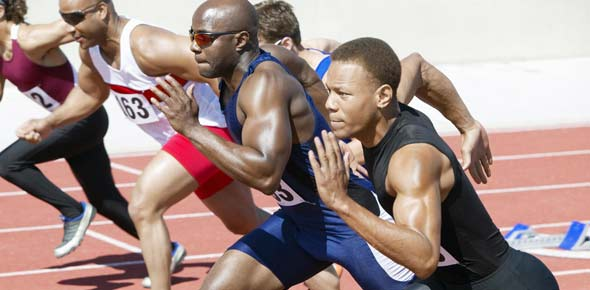PE-7 Track & Field, And Fitness Quiz

15 Questions | Total Attempts: 5620SettingsThis is a 15-question quiz on Track & Field, and Fitness concepts (like Heart Rate, the Cardiovascular System, and Aerobic & Anaerobic Exercise).

• 1.
Which of the following Track & Field Events would be considered Anaerobic?
• A.

Shot Put

• B.

Marathon

• C.

3,000 Meter run

• D.

10,000 Meter run

• E.

None of the above

• 2.
Name 1 of the 4 parts of the Cardiovascular System.
• 3.
In regards to fitness, what does THR stand for?
• 4.
Aerobic Exercise is exercise that takes place over a longer period of time, involves endurance, and is performed with oxygen.  True or False.
• A.

True

• B.

False

• 5.
Anaerobic Exercise is exercise that takes place over a longer period of time, involves endurance, and is performed with oxygen.  True or False.
• A.

True

• B.

False

• 6.
There are 9 (nine) events in the decathlon.  True or False.
• A.

True

• B.

False

• 7.
A short sprint race, like the 100 Meter dash, is a type of Anaerobic exercise.  True or False.
• A.

True

• B.

False

• 8.
The type of baton pass used in the 4x100 Meter relay race is called a ______________ pass.
• 9.
The 4x100 Meter relay race would be considered an Aerobic type of exercise for the 4 members of the relay team.  True or False.
• A.

True

• B.

False

• 10.
Which of the following is the formula used to calculate your MHR?
• A.

200-10 = MHR

• B.

212-12 = MHR

• C.

• D.

• E.

75% of your THR = MHR

• 11.
Which of the following Track and Field events would be a good example of an Aerobic exercise?
• A.

Pole Vault

• B.

Discus

• C.

Hammer Throw

• D.

Marathon

• E.

None of the above

• 12.
In the discus, measurement of how far the discus traveled is made from the end of the platform to where the discus stops rolling or bouncing.  True or False
• A.

True

• B.

False

• 13.
In a relay race, the area in which you pass the baton to your teammate is called the _____________.
• A.

Baton Zone

• B.

Running Zone

• C.

Stick Zone

• D.

Passing Zone

• E.

Twilight Zone

• 14.
For maximum distance, you should throw the shot put like you do a baseball.  True or False.
• A.

True

• B.

False

• 15.
Which of the following are the 3 commands you hear for the start of a race?
• A.

On your mark, get set, go

• B.

On your mark, get set, run

• C.Back to top